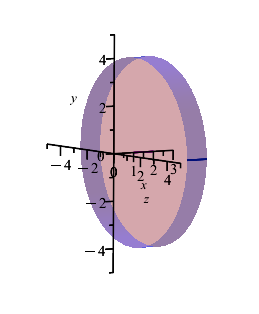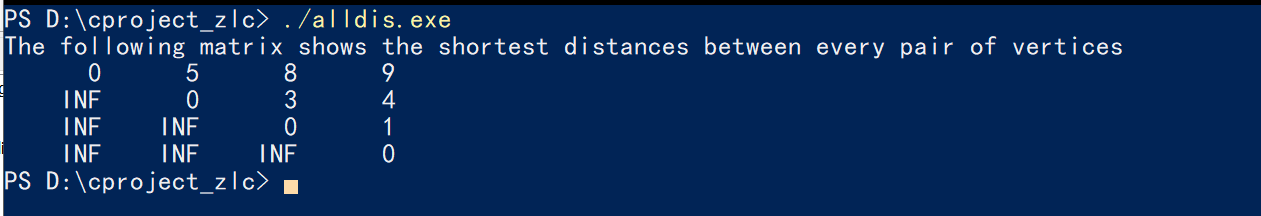## 1) Where to make suggestions for Maple Flow 2022? ...

1 - Is there a specefic place to make suggestions for the next revision of Maple Flow 2022?

(As opposed to just blasting them out on the forum)

2 - When entering text in a container...

I hit Ctl+R to switch to Math mode ... but how do I switch back to text mode in the same container?

For instance I hit the space bar for a text container and enter some text...

Then I hit Ctl+R to enter some math like maybe to enter a variable with a subscript...

Then I want to return to text mode in the same container...

How do I do this?

Thanks for any help.

## How can I attach labels to LP solution matrices an...

Good day.

I am working on a network flow problem that uses a linear programming (simplex) technique.The flow is directed across 3 regions (a, b, and c) and there are 2 nodes within region a, 4 nodes in region b, and 5 nodes in region c.

I wish to determine the minimum cost from regions a to c and from regions b to c that satisfies the requirement of each of the 5 nodes in region c. The objective and constraints are constructed and Maple produces the minimum solution (see attached). While the output is useful when the number of nodes is small, I would like to extend this to a larger-scale case and so the output would need to be more user-friendly / graphic.

I have 2 questions -

1. Does anyone know a way to attach labels for each region and each node to these solution matrices?
For instance -  the first matrix, x, specifies a quantity of 2,000 that flows from node #1 in region a to node #2 in region b
2. Is it possible to produce a network graph showing the directed flow from each region and from node to node?

Thanks to all for reading this!

MaplePrimes_Network.mw

## Fix or reorder terms...

I'm displaying several lines of equations that are all pretty similar and sometimes maple changes the order making it look unpleasent. e.g., the equations are essentially of the form a - b and sometimes maple will output -b + a

If I do sort(E) then it prints them all as -b+a and I cannot get this reverse no matter what options I try

I can do op(E) and it breaks up the expression in to terms but add(op(E)) just ends up doing the same out of order expression.

%+(seq(Reverse([op(E))])));

essentially gives me what I want, surely there is an easier and more robust way?

## VolumeOfRevolution() surface color...

How do you apply colors to an entire solid of revolution? I can't seem to color the bases of a disk. Please help.

Here is what I have. I would like the entire solid to be the same color,transparency,etc.

v := VolumeOfRevolution(4, x = 1 .. 2, volumeoptions = [color = blue, transparency = 0.6], orientation = [270, 0, 15], view = [0 .. 3, -5 .. 5, -5 .. 5], labels = [x, y, z], output = plot, axis = horizontal, scaling = constrained)## Better printing...

I'd like to print a high res and quality worksheet but maple has very poor printing.

I'd like to change the font so I can print more text. I'd like to change the dpi of the print to get higher quality.

Right now I'm having to print screen then scale in photoshop just to get a halfway decent print. I don't want pages of wrapped text that is very confusing to view. If I zoom out all the way on the worksheet I can almost get what I want as far as the size and wrapping(I want to avoid wrapping and there seems to be no way to turn this "feature" off). Of course when I zoom out and use print screen the quality is extremely low due to resolution issues. [So "zooming out" let's me stuff most of the data in to view that I want but then when I use print screen to capture it the quality is so low as not to be useable due to the dpi issue. Of course if I use any of maples printing "features" it will ignore the zoom scaling so as to be useless]

The only thing I can see on how to solve this issue is to save to latex and modify the latex. The problem is that maple puts a lot of junk formatting in the latex to make the wrapping look the same which is exactly what I do not want.

Maple has a "copy as image" but this doesn't seem to do anything. The printing of Maple really needs to be worked on. It's so limited to be almost useless.

## limit and series...

Why solutions (5) and (6) are different? The solution (5) is obtained by putting f=0 of the series, while (6) is the result by taking limit at f->0.

check.mw

## PDEtools declare but still using the standard part...

I want to know if it is possible to use the declare command from the PDETools package for a function

f(x,y,z)

and still, having the notation $\frac{\partial f}{\partial x}$ instead of $f_x$ for the Maple command %diff(f(x,y,z),x)

Thanks !

Kevin

## Getting error too many levels of recursion over th...

 >>(1)
 >(2)
 >(3)
 >(4)
 >(5)
 >(6)
 >>>Error, invalid function arguments>Here psi is a general wave function from schrodinger wave equation.

## Can Maple's functions be packaged as executable fi...

The problem comes from the link https://www.mapleprimes.com/questions/234398-Convert-Maple-Code-To-Python-#comment287424.

We know that when we compile a C or C ++ function, it generates an executable file.  Then we are free from source code.  For example. the function below returns a square matrix A where    "A[i, j]" is the distance from vertex i to vertex j in the graph G. My computer system is Windows.

// C Program for Floyd Warshall Algorithm
#include <stdio.h>

// Number of vertices in the graph
#define V 4

/* Define Infinite as a large enough
value. This value will be used
for vertices not connected to each other */
#define INF 99999

// A function to print the solution matrix
void printSolution(int dist[][V]);

// Solves the all-pairs shortest path
// problem using Floyd Warshall algorithm
void floydWarshall (int graph[][V]) {
/* dist[][] will be the output matrix
that will finally have the shortest
distances between every pair of vertices */
int dist[V][V], i, j, k;

/* Initialize the solution matrix
same as input graph matrix. Or
we can say the initial values of
shortest distances are based
on shortest paths considering no
intermediate vertex. */
for (i = 0; i < V; i++)
for (j = 0; j < V; j++)
dist[i][j] = graph[i][j];

/* Add all vertices one by one to
the set of intermediate vertices.
---> Before start of an iteration, we
have shortest distances between all
pairs of vertices such that the shortest
distances consider only the
vertices in set {0, 1, 2, .. k-1} as
intermediate vertices.
----> After the end of an iteration,
vertex no. k is added to the set of
intermediate vertices and the set
becomes {0, 1, 2, .. k} */
for (k = 0; k < V; k++) {
// Pick all vertices as source one by one
for (i = 0; i < V; i++) {
// Pick all vertices as destination for the
// above picked source
for (j = 0; j < V; j++) {
// If vertex k is on the shortest path from
// i to j, then update the value of dist[i][j]
if (dist[i][k] + dist[k][j] < dist[i][j])
dist[i][j] = dist[i][k] + dist[k][j];
}
}
}

// Print the shortest distance matrix
printSolution(dist);
}

/* A utility function to print solution */
void printSolution(int dist[][V]) {
printf ("The following matrix shows the shortest distances"
" between every pair of vertices \n");
for (int i = 0; i < V; i++) {
for (int j = 0; j < V; j++) {
if (dist[i][j] == INF)
printf("%7s", "INF");
else
printf ("%7d", dist[i][j]);
}
printf("\n");
}
}

// driver program to test above function
int main() {
/* Let us create the following weighted graph
10
(0)------->(3)
|         /|\
5 |          |
|          | 1
\|/         |
(1)------->(2)
3           */
int graph[V][V] = { {0,   5,  INF, 10},
{INF, 0,   3, INF},
{INF, INF, 0,   1},
{INF, INF, INF, 0}
};

// Print the solution
floydWarshall(graph);
return 0;
}

The above functions will be packaged as the disall.exe , and then we will move them anywhere in my computer and run it in Powershell.  We don't have to deal with the source code unless we want to change it.I mean can Maple do something like that?

with(GraphTheory);
G := Graph([1, 2, 3, 4, 5], {{1, 2}, {1, 3}, {1, 4}, {1, 5}, {2, 3}, {2, 5}, {3, 4}, {4, 5}});
AllPairsDistance(G);


For exmaple, can I package the above code snippet into an exe file?

## Maple Flow 2022 Calculation Question...

Given: f := (R1 + Rc)/(4*C*Rf*R1)

Where ...

R1 := 10^5

R2 := 300*10^3

R3 := 75*10^3

Rf := 10^4

Rc := R2

C := 10^(-6)

Now to find the value of "f" enter...

f=

and I get ...

25.000 + 2.500*10^(-4)*Rc

But ...if I enter ...

(R1 + Rc)/(4*C*Rf*R1) =

I get 100.000

Which I think is the correct answer.

Why isn't Maple Flow 2022 returning 100 when I ask for the value of "f" ?

I'm sure I'm doing something incorrect so maybe some kind soul will give me some guidance.

Thanks for any help.

PS - Is there an easy way to enter a set of equations from Maple Flow  2022... like the above... without having to copy and paste each one individually?

## how do i calculate this integral...

hallo evert body please how i do calculate this integral

in maple 18

ttp.pdf

int_{0}^{2\pi}(\cos^{i}(\theta)-\cos^{i+2}(\theta))d\theta
`

## How can I extract the Chebyshev coefficients of th...

Dear All,
I want to extract the coefficients of Chebyshev of an arbitrary function, for example, exp(x). I know that we can use the following command to make a Chebyshev series expansion of exp(x):
chebyshev(exp(x),x);
the above returns the sum of nth Chebyshev polynomials multiplied by Chebyshev coefficients as the following:
1.26606587775201*T(0, x) + 1.13031820798497*T(1, x) + 0.271495339534077*T(2, x) + 0.0443368498486638*T(3, x) + 0.00547424044209371*T(4, x) + 0.000542926311913993*T(5, x) + 0.0000449773229542760*T(6, x) + 3.19843646244580*10^(-6)*T(7, x) + 1.99212480641582*10^(-7)*T(8, x) + 1.10367717095000*10^(-8)*T(9, x) + 5.50589697979079*10^(-10)*T(10, x)

I like to take the coefficients 1.266,1.1303, 0.2714, 0.04433, and so on. How can I do it?
Thanks

## Why GenerateMatrix produces wrong results?...

Why GenerateMatrix produces wrong results?>>>>>>>(1)
 >>(2)
 >>>## How to run programmatically Windows PowerShell com...

I use Maple 2020 on a Windows 10 PC.

The command ssystem("CMD") enables to launch any Windows command accessible from the shell.
But how to launch a PowerShell command?

For instance ssystem("get-process") returns -1 (not surprising in fact for get-process is not a shell process).
How can I tell Maple that this command is to be found in the PowerShell ?

And even, I this possible for, in the ssystem help page, it's said that not all the command can be launched by ssystem

TIA

## Convert maple code to python ...

I have quite a big code with lot of functions how to convert all together to python in one go

Or any other way of advice

As recoding is difficult. Can you help with a small sample program with 2 simple functions returning values

Graph theory and it's algorithms, linear algebra , have used in my program codings mainly

In small function kind take to a create a small graph find allpairshortestpath

It would help to understand how it converts